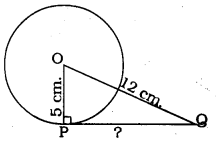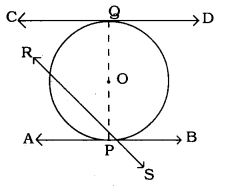# KSEEB SSLC Class 10 Maths Solutions Chapter 4 Circles Ex 4.1

In this chapter, we provide KSEEB SSLC Class 10 Maths Solutions Chapter 4 Circles Ex 4.1 for English medium students, Which will very helpful for every student in their exams. Students can download the latest KSEEB SSLC Class 10 Maths Solutions CChapter 4 Circles Ex 4.1 pdf, free KSEEB SSLC Class 10 Maths Solutions Chapter 4 Circles Ex 4.1 book pdf download. Now you will get step by step solution to each question.

### Karnataka State Syllabus Class 10 Maths Solutions Chapter 4 Circles Ex 4.1

Question 1.
How many tangents can a circle have?
Solution:
There is only one tangent at a point of the circle.

Question 2.
Fill in the blanks :

1. A tangent to a circle intersects it in __________ point (s).
2. A line intersecting a circle in two points is called a ____________
3. A circle can have _____________ parallel tangents at the most
4. The common point of a tangent to a circle and the circle is called _____________ .

1. one
2. tangent
3. two
4. point of contact.

Question 3.
A tangent PQ at a point P of a circle of radius 5 cm meets a line through the centre O at a point Q so that OQ =12 cm, Length PQ is.:
a) 12 cm.
b) 13 cm.
c) 8.5 cm.
d) 19 cm.
Solution:
d) 119−−−√cm.A tangent PQ at a point P of a circle.
OP is the radius drawn from centre of the circle to the tangent.
∴ OP ⊥ PQ.
Now, in ⊥ ∆OPQ, ∠P = 90°
∴ As per Pythagoras theorem,
OP2 + PQ2 = OQ2
(5)2 + PQ2 = (12)2
25 + PQ2 = 144
PQ2 = 144 – 25
PQ2 = 119
∴ √PQ = √119
∴ PQ = √119 cm.

Question 4.
Draw a circle and two lines parallel to a given line such that one is a tangent and the other, a secant to the circle.
Solution:CD is the tangent parallel to AB.
AB is secant, it meets RS at P.

All Chapter KSEEB Solutions For Class 10 Maths

—————————————————————————–

All Subject KSEEB Solutions For Class 10

*************************************************

I think you got complete solutions for this chapter. If You have any queries regarding this chapter, please comment on the below section our subject teacher will answer you. We tried our best to give complete solutions so you got good marks in your exam.

If these solutions have helped you, you can also share kseebsolutionsfor.com to your friends.

Best of Luck!!